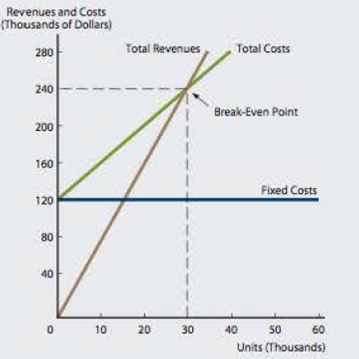Chapter 13, Problem 10P### Fundamentals of Financial Manageme...

9th Edition
Eugene F. Brigham + 1 other
ISBN: 9781305635937

#### Solutions

Chapter
Section### Fundamentals of Financial Manageme...

9th Edition
Eugene F. Brigham + 1 other
ISBN: 9781305635937
Textbook Problem
117 views

# BREAKEVEN AND OPERATING LEVERAGE a. Given the following graphs, calculate the total fixed costs, variable costs per unit, and sales price for Firm A. Firm B's fixed costs are $120,000, its variable costs per unit are$4, and its sales price is $8 per unit. b. Which firm has the higher operating leverage at any given level of sales? Explain. c. At what sales level, in units, do both firms earn the same operating profit? Firm AFirm Ba. Summary Introduction To identify: The fixed cost, variable cost per unit and sales price for Firm A. Introduction: Cost-Volume-Profit Analysis: The cost-volume analysis is the method to analyze the net income of the company with the help of fixed cost, variable cost, and sales volume. The change in the sales volume results to a change in fixed cost and variable cost with an effect to the net income. Explanation Compute the fixed cost. The fixed cost is shown from the horizontal line of the graph that is$80,000. Hence, the fixed cost of the firm is $80,000. Compute the variable cost per unit. Given, The total cost is$200,000.

The fixed cost is $80,000. Formula to calculate the variable cost per unit, Variablecostperunit=TotalcostFixedcostSoldunits Substitute$200,000 for total cost, $80,000 for fixed cost and 25,000 units for sold units. Variablecostperunit=$200,000$80,00025,000units=$120,00025,000units=\$4

b.

Summary Introduction

To identify: The firm with the higher operating leverage.

Introduction:

Operating Leverage: Operating leverage refers to the measure to analyze the effect of change in the sale on operating income

c.

Summary Introduction

To identify: The level of sales, sales units at which the firms have the same operating profit.

Introduction:

Operating Profit: An operating profit refers to the profit which is derived from the deduction of fixed and variable cost from the sales revenue.

### Still sussing out bartleby?

Check out a sample textbook solution.

See a sample solution

#### The Solution to Your Study Problems

Bartleby provides explanations to thousands of textbook problems written by our experts, many with advanced degrees!

Get Started